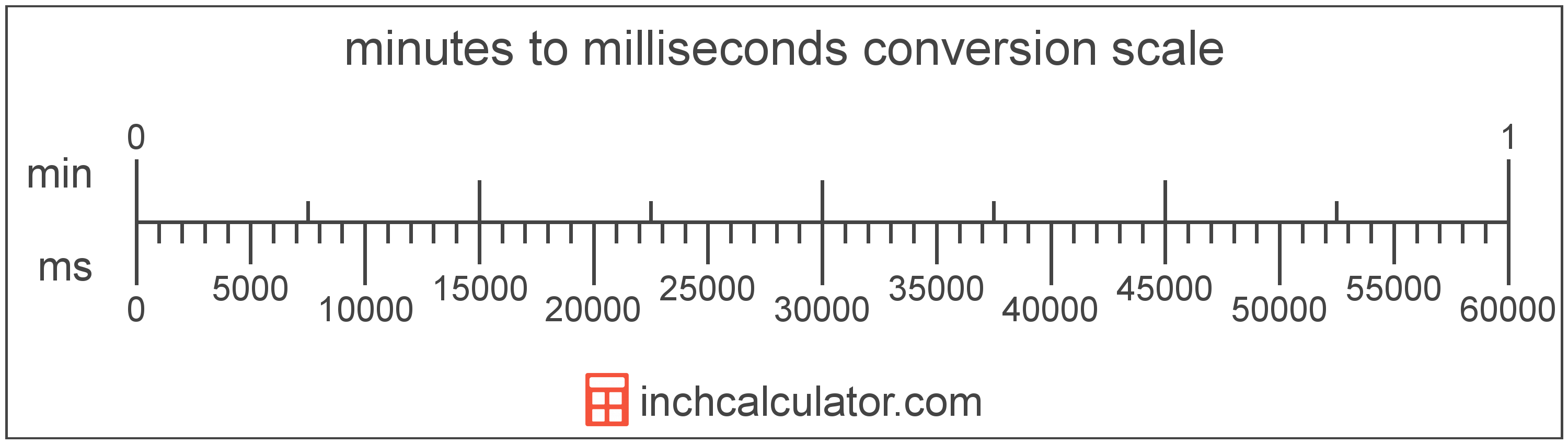# Milliseconds to Minutes Conversion

Enter the time in milliseconds below to get the value converted to minutes.

Results in Minutes:1 ms = 1.6667E-5 min
Hint: use a scientific notation calculator to convert E notation to decimal

## How to Convert Milliseconds to MinutesTo convert a millisecond measurement to a minute measurement, divide the time by the conversion ratio.

Since one minute is equal to 60,000 milliseconds, you can use this simple formula to convert:

minutes = milliseconds ÷ 60,000

The time in minutes is equal to the milliseconds divided by 60,000.

For example, here's how to convert 50,000 milliseconds to minutes using the formula above.
50,000 ms = (50,000 ÷ 60,000) = 0.833333 min

## Milliseconds

The millisecond is a multiple of the second, which is the SI base unit for time. In the metric system, "milli" is the prefix for 10-3. Milliseconds can be abbreviated as ms; for example, 1 millisecond can be written as 1 ms.

## Minutes

The minute is a period of time equal to 1/60 of an hour or 60 seconds.

The minute is an SI accepted unit for time for use with the metric system. Minutes can be abbreviated as min; for example, 1 minute can be written as 1 min.

## Millisecond to Minute Conversion Table

Millisecond measurements converted to minutes
Milliseconds Minutes
1 ms 0.000016667 min
2 ms 0.000033333 min
3 ms 0.00005 min
4 ms 0.000066667 min
5 ms 0.000083333 min
6 ms 0.0001 min
7 ms 0.000117 min
8 ms 0.000133 min
9 ms 0.00015 min
10 ms 0.000167 min
100 ms 0.001667 min
1,000 ms 0.016667 min
10,000 ms 0.166667 min
100,000 ms 1.6667 min